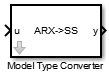# Model Type Converter

Convert polynomial model coefficients to state-space model matrices

## Library

Estimators

•## Description

Use the Model Type Converter block to convert the ARX, ARMAX, OE, or BJ model coefficients into state-space model matrices.

The block inport, u, requires a bus. The number of elements depends on the input polynomial model type:

• ARX — A, B

• ARMAX — A, B, C

• OE — B, F

• BJ — B,C, D, F

These bus elements must contain row vectors of the estimated coefficient values as outputted by the Recursive Polynomial Model Estimator block. For MISO data, specify B polynomial coefficients as a matrix where the i-th row parameters correspond to the i-th input. The coefficient values can vary with time. The Model Type Converter block converts these coefficients into the A, B, C, and D matrices of a discrete-time state-space model. The Model Type Converter block outport, y, returns a bus with elements that correspond to the A, B, C, and D matrices of the state-space model. If the signals in u are time-varying, then the state-space matrices are time-varying too.

You can also estimate a state-space model online by using the Recursive Polynomial Model Estimator and Model Type Converter blocks together. Connect the outport of the Recursive Polynomial Model Estimator block to the inport of the Model Type Converter block to obtain online values of the state-space matrices. The conversion ignores the noise component of the models. In other words, the state-space matrices only capture the y(t)/u(t) relationship.

## Parameters

### Input model type

Specify the model type coefficients to convert to state-space model matrices. Specify one of the following model types:

• `ARX`

• `ARMAX`

• `OE`

• `BJ`

## Ports

Port

Port Type

(In/Out)

Description
uInEstimated A, B, C, D and F polynomial coefficients, specified as a bus with elements: A, B, C, D and F.
yOutState-space model, returned as a bus with elements that correspond to the A, B, C, and D matrices.

## Supported Data Types

• Double-precision floating point

• Single-precision floating point

## Extended Capabilities

### PLC Code GenerationGenerate Structured Text code using Simulink® PLC Coder™.